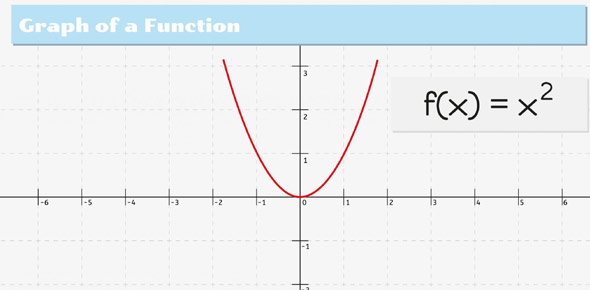# Functions Warm-up

5 Questions | Total Attempts: 86SettingsIn mathematics, a function is an equation that has only one answer for y for every x. A function assigns exactly one output to each input of a specified type. The quiz below is set u to help you in get a deeper understanding on functions. Give it a try and share your results!

Related Topics
• 1.
Which graph represents a function?
• A.

Option 1

• B.

Option 2

• C.

Option 3

• D.

Option 4

• 2.
If g(x) = x2 + 3x - 2, find g(-2).
• A.

-4

• B.

-12

• C.

8

• D.

4

• 3.
Determine which one of these relations is a function.
• A.

Option 1

• B.

Option 2

• C.

Option 3

• D.

Option 4

• 4.
If f(x)=3x+5, find f(2).
• A.

10

• B.

8

• C.

11

• D.

2f

• 5.
Make a table showing the cost of buying 1 to 5 CDs if CDs cost \$12 each.
• A.

Option 1

• B.

Option 2

• C.

Option 3

• D.

Option 4